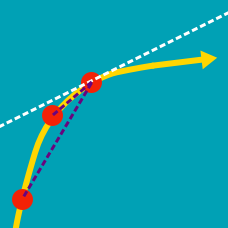Calculus

# Derivatives of Linear Functions

If $f(x)=8x+\frac{7}{19},$ what is the value of $f'(x)?$

If $f(x)=11x-17,$ what is $f'(5)?$

If $f(x)$ is a linear function and $f'(-11)=17,$ what is the value of $f'(11)?$

If $f(x)$ is a linear function with $f(5)=60$ and $f'(5)=3,$ what is the value of $f(-3)+f'(-3)?$

If $f(x)$ and $g(x)$ are two linear functions with $f'(1)=-5$ and $g'(1)=8,$ what is the value of the derivative of the function $f(x)-2g(x)$ at $x=1?$

×